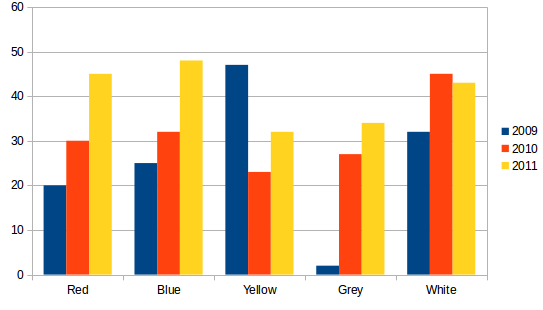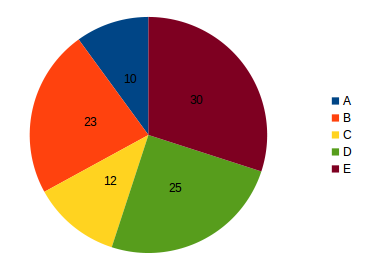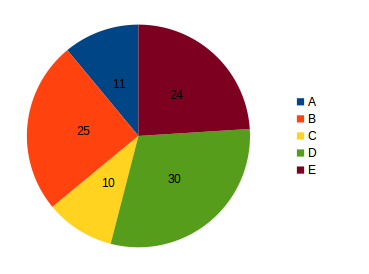## Data Interpretation For SBI PO : Set – 58

D.1-4) Study the graph of Sales of Different Colours of Brand X Refrigerator for the years 2009,2010 and 2011 to answer these questions.1) Over the given three years which has been the highest selling colour and what percentage of Volume did it account for?

a) Blue, 21%

b) White, 25%

c) White, 25%

d) Yellow, 20%

e) None of these

b)

Over the given 3 years sales volume of red colour = (20+30+45)=95 thousand

Sales Volume of blue colour =(25+32+48)=105 thousand

Sales volume of Yellow colour =(47+23+32)=102 thousand

Sales Volume of grey colour = (2+27+34)=63 thousand

Sales Volume of white colour =(32+45+43)=120 thousand

Thus, white is the highest selling colour and its percentage volume

120/(95+105+102+63+120)*100= 12000/485=25%

2) Sale of which colour of refrigerator registered the highest percentage increase between 2010 and 2011?

a) Blue

b) Lemon

c) Red and Blue

d) Grey

e) None of these

c)

Percentage increase in sales between 2010 and 2011 for blue = (48-32/32)*100=50%

For yellow = (32-23)/23*100=39%

For red = (45-30)/30*100=50%

For grey = (34-27)/27*100=26%

Thus red and blue registered the highest percentage increase

3) What is the share of sales volume accounted for by the year 2011?

a) 43.26%

b) 46.14%

c) 44.16%

d) 41.64%

e) None of these

d)

Sales Volume in  2011 = (45+48+32+34+43)= 202 thousand

Total Sales Volume in all the 3 year = 485 thousand

Required Percentage = 202/485*100=41.64

4) What is approximately the ratio of sales of Red, yellow and White refrigerators taken together in 2010 to that of the combined total sales of blue and Green ones in 2009 and 2011 taken together?

a) 9:10

b) 10:9

c) 11:12

d) 1:1.2

e) None of these

a)

Sales Volume of red, yellow and white refrigerators in 2010 = (30+23+45)=98 thousand

Sales Volume of Blue and grey in 2009 and 2011 =(25+48+2+34)=109 thousand

Required ratio = 98:109 = 9:10

D.5-10) Refer to the pie charts and answer the given questions.

The following data are regarding number of students (boy+girls) studying in Standard X th of various schools (A,B,C,D and E in the year 2010)

Total Number of Students (Boys+Girls) = 3000Total Number of Boys = 30005) what is the difference between the total number of students(boys+girls) in Schools A and B together and the total number of Students (boy+girls) in Schoold D and E together?

a) 640

b) 600

c) 620

d) 680

e) 660

e)

Total Number of Boys and girls in School (A+B) =[(176+400)]+[(124+290)]=576+414=990

Total Number of boys and girls in School (D+E) [(480+384)]=[(270+516)]=864+786=1650

Required difference =1650-990= 660

6) Number of girls studying in School C, are what percent of total number of students(boys+girls) studying in the same school?

a) 48

b) 60 1/3

c) 50

d) 55 5/9

e) 42 5/8

d)

Number of boys in school C = 1600*10/100=160

Number of girls in School C = 3000*12/100-160=360-160=200

Total Number of students in school C = 3000*12/100=360

Required Percentage = (200/360)*100=500/9=55 5/9%

7) What is the average number of boys studying in School A and B?

a) 280

b) 288

c) 272

d) 248

e) 278

b)

Number of boys in School A = 1600*11/100=176

Number of boys in school B = 1600*25/100=400

Average number of boys in School A and B = 176+400/2 = 576/2 = 288

8) Number of girl studying in School E are approximately what percent more than the number of boys studying in the same school?

a) 45%

b) 30%

c) 50%

d) 40%

e) 35%

e)

Number of boys in school E = 1600*24/100=384

Number of girls in School E = (3000*30/100-384)=900-384=516

Required Percentage (516/384)*100-100)%=(134.375-100)%=34.375%=35%

9) What is the respective ratio between the number of boys and the number of girls studying in School D?

a) 12:7

b) 16:9

c) 16:7

d) 8:7

e) 14:9

d)

Number of boys in school d = 1600*30/100=480

Number of girls in School D =(3000*30/100-480)=900-480=420

Required ratio = 480:420=8:7

10) what is the Total number of boys studying in a school B and C?

a) 200

b) 500

c) 880

d) 800

e) None of these